soft drawing: lines and ellipsesdownload demo programlook at source codedownload Delphi-7 project

Drawing of soft lines and ellipsesAbove left you see a picture from the demo program, right the left top 10 fold enlarged.

The "soft" shapes are obtained by spreading the pen over the pixel boundaries.
The image below clearly demonstrates the difference.
The top line is "soft", the line below shows staircases because the positions are rounded to pixel accuracy.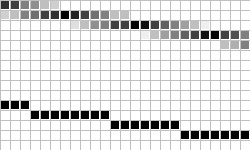The theory: lines

Distinguished are horizontal- and vertical oriented lines.
Horizontal lines have a tangent of -45..45 degrees,
the vertically oriented lines have tangents of -90..-45 or 45..90 degrees.
Pixels are regarded as a square of 4*4 subpixels.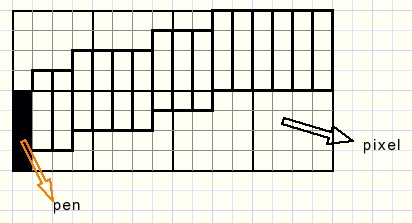Pictured are 2 rows of each 4 pixels.
In horizontal oriented lines the pen (black) has a width of 0.25 and a height of 1 pixel.
The drawing process moves the pen right by steps of 0.25 pixel.
The related y value is calculated and rounded to a multiple of 0.25

A vertically oriented line looks like this: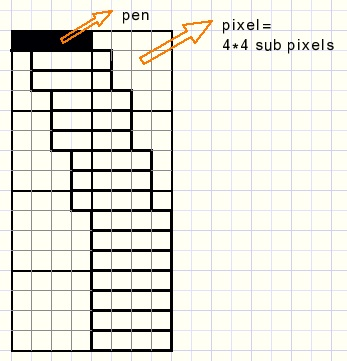The (black) pen is 1 pixel wide and 0.25 pixel of height.
The drawing process increases y by steps of 0.25 and the related x value is calculated
and rounded to a multiple of 0.25

The drawing is made visible by placing it on the canvas of a paintbox.
Directly drawing on the paintbox is not advisable because it is a very slow process.
Drawing on a bitmap is much faster.
After drawing, the bitmap has to be copied to the paintbox to become visible.

A problem at this point is that we have to paint the pen on 4 subpixels,
which may belong to two pixels.
Also, for each pixel we have to register which subpixels were colored by the pen.
For this reason there is a 2-dimensional array of words, called blender.
Each bit in a word of blender corresponds with a subpixel which receives the value "1" when colored.
The blender array has the same dimensions as the bitmap.

The total drawing process takes three steps:
1. bits in array blender are set to "1" if covered by the pen
2. the blender array is scanned and the bitmap pixel colors are calculated.
3. the bitmap is copied to a paintbox to become visible.
step 2 :
The number of "1" bits in a word determines the influence of the pen.
At a value of 16 the pixel receives the pen color.
At a value of 5, the final pixel color is a blend of 5/16 pencolor and 11/16 pixelcolor.
Below you see the relation between blender word bits and the subpixels: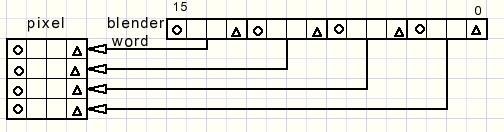The program

The program is centered around two procedures:
1. procedure Qpixel(x,y : single);
2. procedure procblendRect;

1.
The parameters (x,y) are of type single, which enables crossing of the pixel boundaries.
Different pen shapes are selected for horizontal- and vertical oriented lines.
The procedure paints a quarter pixel at (x,y).

2.
Rectangle blendRect holds the area of change by the last drawing action.
ProcBlendRect scans the words in this rectangle and modifies the bitmap pixels if necessary.

Please refer to the source code for details.

Procedure Softline(x1,y1,x2,y2 : smallInt) draws the line from (x1,y1) to (x2,y2).
Qpixel is called repeatedly followed finally by procBlendRect.
The pen always moves left to right.
If x1 > x2 than the coordinates are traded before drawing starts.

Ellipses

Procedure SoftEllipse(x1,y1,x,y2 : smallInt) draws a soft ellipse within rectangle (x1,y1) --> (x2,y2).
QPixel is called repeatedly, finally followed by procBlendRect, the same as for lines.

Additional problem is the pen selection, which depends on the line direction.
The ellipse has to be divided into horizontal and vertical (oriented) arcs, two of each.
The border between these arcs is the point where the ellipse tangent = -1.

The general equation of an ellipse having length 2a and height 2b is
(x/a)2 + (y/b)2 = 1
Remember: a circle having radius=1 has the equation : x2+y2= 1
Stretching the circle horizontally by a factor a and vertically by b results in the ellipse.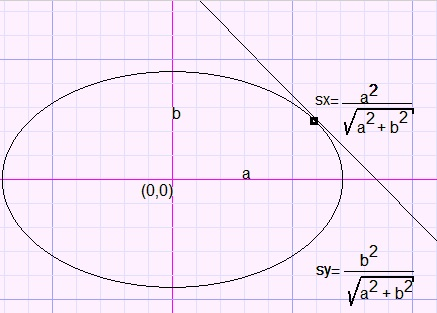(sx,sy) is the pivot point between the horizontal and the vertical orientation.

The ellips has center coordinates (0,0).
From this point x is increased and decreased by 0.25 until sx or -sx is reached.
For the vertical parts y is increased and decreased by -.25 until sy or -sy is reached.
In each case he corresponding y or x value is calculated and Qpixels(..,..) is called.

This concludes the softdraw description.
Please refer to the source code for more details.